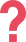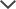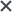If you have any questions, feel free to contact Eitan — TestPrep-Online's CAT4 expert — at ask_eitan@testprep-online.com

## About the CAT4 Level F

The Fourth Edition Cognitive Abilities Test (CAT4) is an exam developed by GL Assessment to help schools understand their students' abilities and academic potential. By monitoring the students' performance on the test, teachers can use the test results to identify individual strengths and weaknesses and make implications to the school's studying process. The test is comprised of the following sections:

• Quantitative Reasoning-Number Series and Number Analogies
• Verbal Reasoning-Verbal Classification and Verbal Analogies
• Non-Verbal Reasoning-Figure Classification and Figure Matrices
• Spatial Ability-Figure Analysis and Figure Recognition

the CAT4 has also become widely common as an international admission tool for luxurious schools around the world. Level F of the CAT4 exam is designated for UK's year nine and year ten (Y9 & Y10), catering to children between 12 and 15.

## CAT4 Level F Sample Questions

 CAT4 Level F Test Practice Question-Quantitative Reasoning #1 What number comes next? 75     72     69     74     71     68     73     70     ? 64 65 67 73 75 Answer & Explanation ▼ | ▲ The correct answer is 67. In this series, the pattern is as follows: First 3 is subtracted, then 3 is subtracted again, then 5 is added, then 3 is subtracted, then 3 is subtracted again, then 5 is added, and so on. The pattern is: -3, -3, +5, - 3, -3, +5 … The last three numbers are 68, 73, and 70. 73 is 68 added by 5, and 70 is 73 subtracted by 3. According to the pattern, after one subtraction of 3 there is another subtraction of 3. Thus, the next number in the series will be the result of subtracting 3 from 70: 70 - 3 = 67. Therefore, 67 is the correct answer. #Note that additional practice questions for the Quantitative Reasoning battery (number analogies + number series) can be found on our CAT4 Level F practice packs.
 CAT4 Level F Test Practice Question-Verbal Reasoning #1 Helmet → injury : vaccination → medicine disease inoculation surgeon accident Answer & Explanation ▼ | ▲ The correct answer is: disease. Helmets protect from injuries as vaccinations protect from diseases. #Note that additional practice questions for the Verbal Reasoning battery (Verbal analogies + Verbal classification) can be found on our CAT4 Level F practice packs.

CAT4 Level F Test Practice Question-non verbal reasoning#1The correct answer is 4.

In the bottom row we should have the same relationship. We can eliminate the 3rd answer choice because it contains a grey heart and not a white heart. We can also eliminate the 1st and 5th choices because they contain frowny faces and not smiley faces. Finally we can eliminate the 2nd answer choice because we should have a smiley face in the upper-right corner (where there was a down arrow in the left frame), and a sun in the upper-left and lower-right corners (where in the left frame we had up arrows), but we have the opposite. We are left with the 4th choice as the only correct answer.

#Note that additional practice questions for the Non-Verbal Reasoning battery (Figure analogies + Figure classification) can be found on our CAT4 Level F practice packs.

CAT4 Level F Test Practice Question-spatial ability#1The correct answer is (E).

The given shape contains diagonal lines and two parallel horizontal lines.

Answer (A) is missing a line segment to form the top vertex in order to form the given shape, and therefore it can be ruled out.Answer (B) includes a pair of horizontal lines but is missing a vertex on the right in order to form the correct shape, making it also incorrect.Answer (C) has two parallel horizontal lines, however they are too far apart to form the given shape, thus it is eliminated.

Answer (D) includes a shape similar to the given shape, however its left side has a different angle than the left side of the given shape (As shown in the picture below). In addition, the vertex on the right has a different angle than the one in the given shape. Therefore, it does not include the given shape and it is also incorrect.

#Note that additional practice questions for the Spatial Ability battery (Paper folding + Figure recognition) can be found on our CAT4 Level F practice packs.## CAT4 Test Format & Sections

The CAT4 exam consists of four test batteries, each of which is designated for examining different aspects of the student's cognitive ability. Each battery is comprised of two short sub-tests that include various types of questions, evaluating the student's ability in distinct ways:

 Verbal Reasoning Verbal ClassificationVerbal Analogies Quantitative Reasoning Number AnalogiesNumber Series Non-verbal Reasoning Figure ClassificationFigure Matrices Spatial Ability Figure AnalysisFigure Recognition

The full CAT4 test is administered in three parts, splitting the quantitative reasoning sub-tests between part 2 and part 3:

 Sub-Test Question Number Test Time Part 1 Figure Classification 24 questions 10 minutes Figure Matrices 24 questions 10 minutes Part 2 Verbal Classification 24 questions 8 minutes Verbal Analogies 24 questions 8 minutes Number Analogies 18 questions 10 minutes Part 3 Number Series 18 questions 8 minutes Figure Analysis 18 questions 9 minutes Figure Recognition 18 questions 9 minutes

## CAT4 Level F Online Test Practice

Our comprehensive Practice Packs include full-length CAT4 practice tests designed to simulate the actual exam by introducing the test's unique concepts and structure. These packs also feature multiple additional practice drills for each of the various sections of the CAT4, alongside an elaborated study guide for all different question types that appear on the test.

All practice materials are administered in a user-friendly online format. Upon finishing each practice test, you will be presented with an extensive score report that allows you to review your performance, track your progress along the studying process, and determine where to focus your efforts based on identifying your strengths and weaknesses.You will also find complete solutions to all questions and read thorough explanations on how to solve each question, together with helpful solving tips that will help you face similar challenges on the upcoming test.

Not sure which CAT4 prep pack will provide the best preparation for your child? feel free to contact Eitan — TestPrep-Online's CAT4 expert — at ask_eitan@testprep-online.com

The CAT4, CogAT, and other trademarks are the property of their respective trademark holders. None of the trademark holders are affiliated with TestPrep-Online or this website.

Need HelpNeed Help